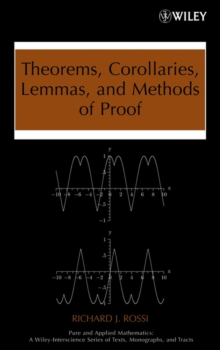Supporting your high street Find out how »
• My AccountTheorems, Corollaries, Lemmas, and Methods of Proof Hardback

Part of the Pure and Applied Mathematics: A Wiley Series of Texts, Monographs and Tracts series

Description

A hands-on introduction to the tools needed for rigorous and theoretical mathematical reasoning Successfully addressing the frustration many students experience as they make the transition from computational mathematics to advanced calculus and algebraic structures, Theorems, Corollaries, Lemmas, and Methods of Proof equips students with the tools needed to succeed while providing a firm foundation in the axiomatic structure of modern mathematics. This essential book: Clearly explains the relationship between definitions, conjectures, theorems, corollaries, lemmas, and proofs Reinforces the foundations of calculus and algebra Explores how to use both a direct and indirect proof to prove a theorem Presents the basic properties of real numbers Discusses how to use mathematical induction to prove a theorem Identifies the different types of theorems Explains how to write a clear and understandable proof Covers the basic structure of modern mathematics and the key components of modern mathematics A complete chapter is dedicated to the different methods of proof such as forward direct proofs, proof by contrapositive, proof by contradiction, mathematical induction, and existence proofs.

In addition, the author has supplied many clear and detailed algorithms that outline these proofs. Theorems, Corollaries, Lemmas, and Methods of Proof uniquely introduces scratch work as an indispensable part of the proof process, encouraging students to use scratch work and creative thinking as the first steps in their attempt to prove a theorem. Once their scratch work successfully demonstrates the truth of the theorem, the proof can be written in a clear and concise fashion.

The basic structure of modern mathematics is discussed, and each of the key components of modern mathematics is defined.

Numerous exercises are included in each chapter, covering a wide range of topics with varied levels of difficulty. Intended as a main text for mathematics courses such as Methods of Proof, Transitions to Advanced Mathematics, and Foundations of Mathematics, the book may also be used as a supplementary textbook in junior- and senior-level courses on advanced calculus, real analysis, and modern algebra.

Information

• Format: Hardback
• Pages: 318 pages
• Publisher: John Wiley & Sons Inc
• Publication Date:
• Category: Mathematical logic
• ISBN: 9780470042953

£118.00

£104.39

on all orders

Pick up orders

from local bookshops

£94.95

£79.65

£127.00

£112.65